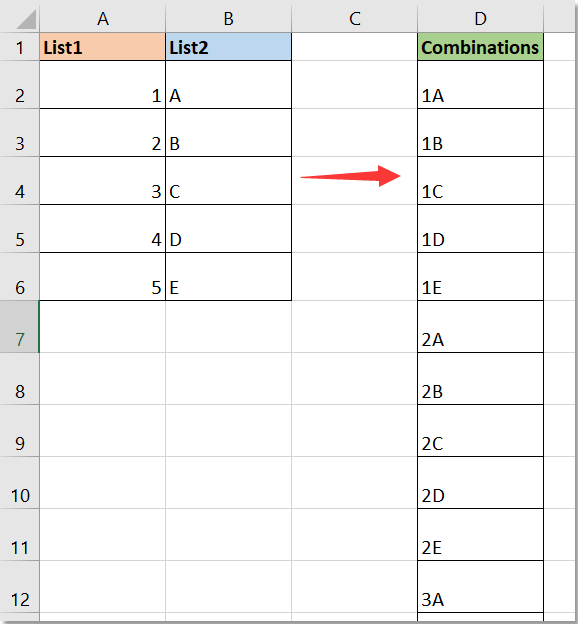## How to find all possible sums of two numbers in a list in Excel?

Supposing here is a list of numbers, now could you find all possible sums of two numbers in this list as below screenshot shown? In Excel, there is no built-in function can solve this job. Now I introduce you a VBA code to handle it.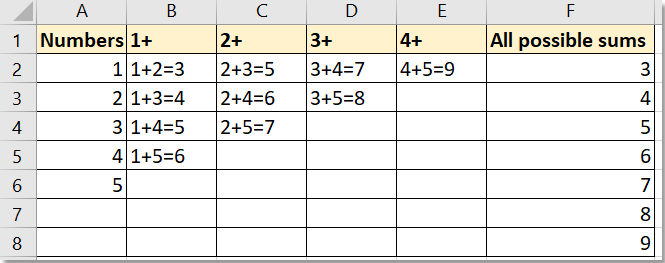Find all possible sums of two numbers in a list with VBA code

#### Find all possible sums of two numbers in a list with VBA code

If you want to find all possible sums of two numbers in a list, you can apply below VBA code.

1. Press Alt + F11 keys to display the Microsoft Visual Basic for Applications window.

2. Click Insert > Module, copy and paste below code to the module.

VBA: List all possible sums of two numbers in a list

``````Sub Combinations()
'UpdatebyExtendoffice20180628
Dim xRgArr
Dim xNum As Long
Dim I, J, K As Long
Dim xTemp As Double
Dim xRg As Range
Dim xRgCount As Long
Dim xDic As New Dictionary
On Error Resume Next
Set xRg = Application.InputBox("Select a list (one column):", "KuTools for Excel", Selection.Address, , , , , 8)
If (xRg Is Nothing) Or (xRg.Count = 1) Then Exit Sub
xRgCount = xRg.Count
K = 1
ReDim xRgArr(1 To xRgCount)
For Each xCell In xRg
xRgArr(K) = xCell.Value
K = K + 1
Next
K = 0
For I = 1 To xRgCount
For J = I + 1 To xRgCount
xTemp = xRgArr(I) + xRgArr(J)
If Not xDic.Exists(xTemp) Then
K = K + 1
End If
Next
Next
Range("C1").Resize(xDic.Count, 1) = Application.WorksheetFunction.Transpose(xDic.Keys)
End Sub``````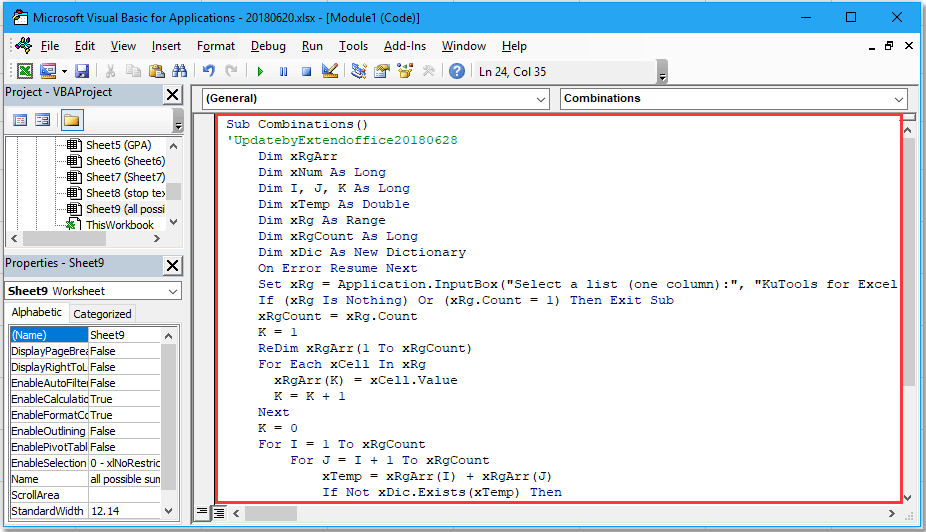3. Click Tools > References, in the popping dialog, check Microsoft Scripting Runtime checkbox.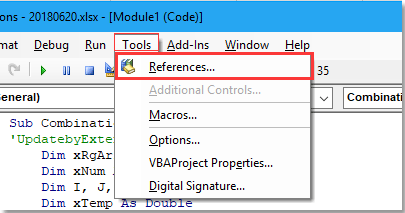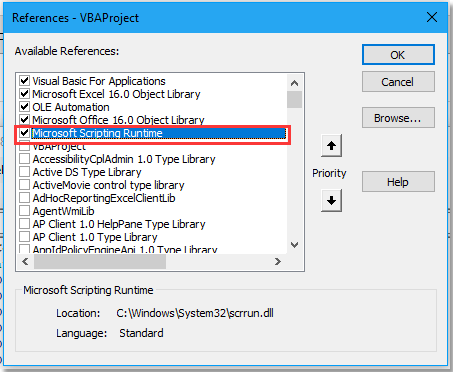4. Click OK to close the dialog, then press F5 to run the code, a dialog pops out to remind you selecting a list to find the possible sums.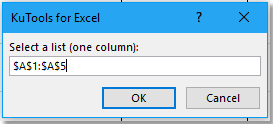5. Click OK, then all possible sums of two numbers in the list are listed in column C.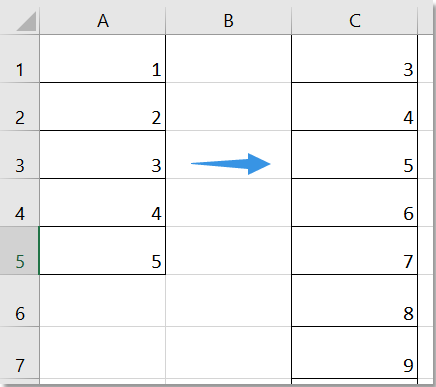Tip：in the code string, Range("C1").Resize(xDic.Count, 1), you can change C1 to another cell as you need to place the result.

#### Find all possible combinations of two lists with List All Combinations

If you want to find or list all possible combinations of two lists as below screenshot shown, the List All Combinations utility of Kutools for Excel can do you a favor.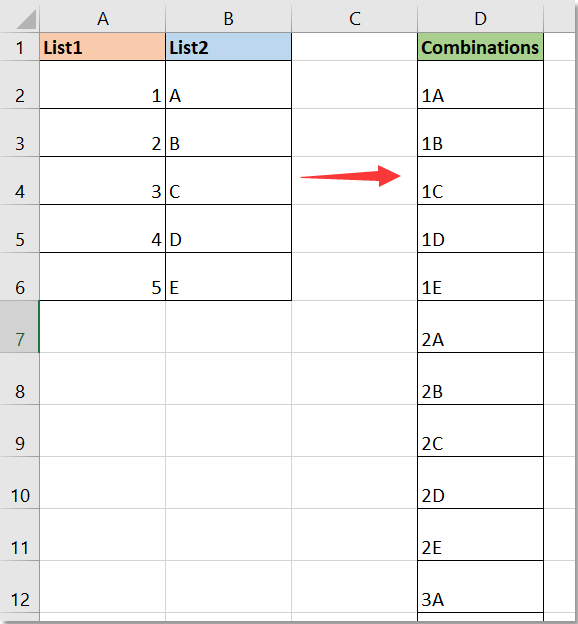with more than 300 handy functions, makes your jobs more easier. Free Downloadfree full-featured in 30 days

1. Click Kutools > Insert > List All Combinations.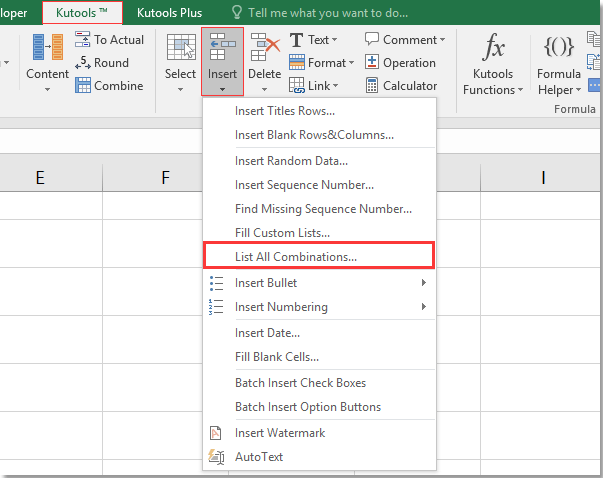2. In the List All Combinations dialog, click the select button to select the first list you will combine.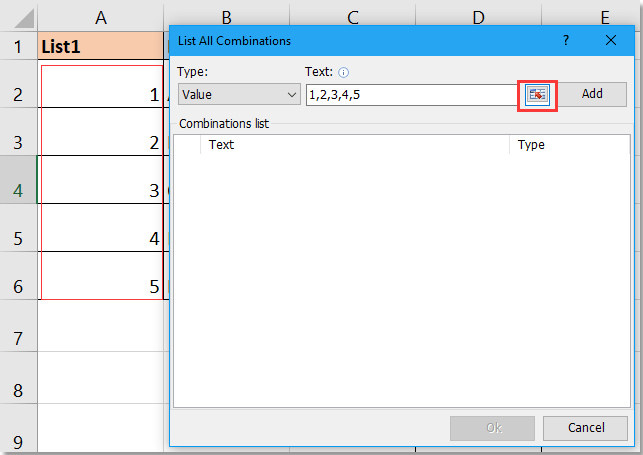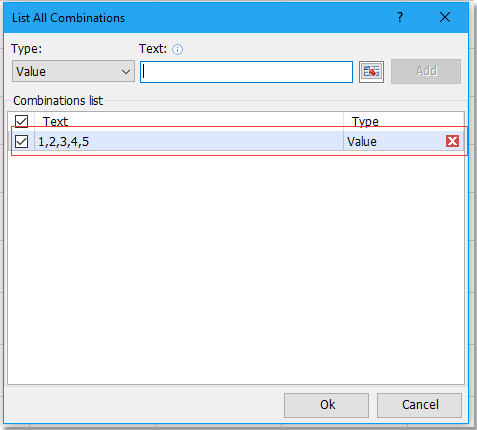4. Repeat step 2 and 3 to add the second list to the Combinations list.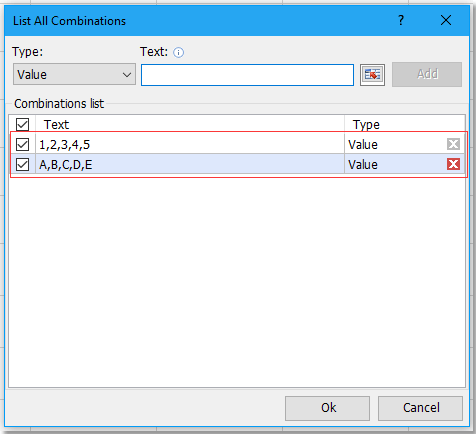5. Then click Ok to select a cell to place the combination result.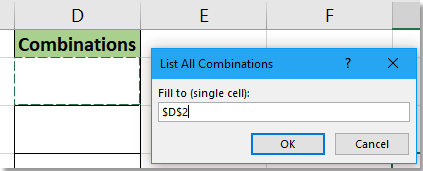6. Click OK. All possible combinations have been listed in cells.